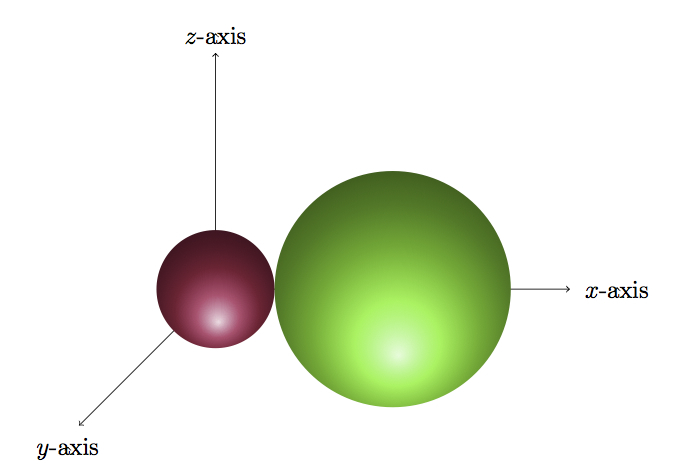# Global Positioning System I

Alignments to Content Standards: A-CED.A.2 G-GMD.B.4

The distance between two points $(x_1,y_1,z_1)$ and $(x_2,y_2,z_2)$ is given by $$\sqrt{(x_1 - x_2)^2 + (y_1-y_2)^2 + (z_1-z_2)^2}.$$

1. For each of the following equations, describe the set of solutions geometrically and sketch this solution set in $x$-$y$-$z$ coordinates:
1. $x^2 + y^2 + z^2 = 1$.
2. $(x-3)^2 + y^2 + z^2 = 4$.
3. $x^2 + y^2 + \left(z- \frac{3}{2}\right)^2 = 1$.
2. Find the set of real numbers $(x,y,z)$ which are solutions both to $x^2 + y^2 + z^2 = 1$ and to $(x-3)^2 + y^2 + z^2 = 4$. Sketch this solution set in $x$-$y$-$z$ coordinates.
3. Find the set of real numbers $(x,y,z)$ which are solutions to both $x^2 + y^2 + z^2 = 1$ and $x^2 + y^2 + \left(z - \frac{3}{2}\right)^2 = 1$. Sketch the solution set in $x$-$y$-$z$ coordinates.

## IM Commentary

This question examines the algebraic equations for three different spheres. The intersections of each pair of spheres are then studied, both using the equations and thinking about the geometry of the spheres. For two spheres where one is not contained inside of the other there are three possibilities for how they intersect:

• The two spheres do not meet at all: this happens if the sum of the radii of the two spheres in question is less than the distance between their centers. This is true for the spheres given by the equations $x^2 + y^2 + \left(z - \frac{3}{2}\right)^2 = 1$ and $(x-3)^2 + y^2 + z^2 = 4$. Though not prompted in the question, the teacher may wish to discuss this fact.
• The spheres meet in exactly one point: this happens if the sum of the radii of the two spheres is equal to the distance between their centers. This is true for the spheres given by the equations $x^2 + y^2 + z^2 = 1$ and $(x-3)^2 + y^2 + z^2 = 4$.
• The spheres meet in a circle when the sum of the radii is greater than the distance between the two centers. This is true for the spheres given by the equations $x^2 + y^2 + z^2 = 1$ and $x^2 + y^2 + \left(z - \frac{3}{2}\right)^2 = 1$.

Teachers should stress two different approaches to this problem: a visual approach and an algebraic approach. The equations for these spheres have been chosen in such a fashion as to make solving the pairs of equations algebraically feasible but the intuition behind the algebra would be greatly aided and assisted by having a geometric picture in mind.

Although only a couple of examples are considered here, it is not too hard to extrapolate from here to deal with equations of general spheres. By choosing coordinates appropriately one of the spheres may be centered at the origin $(0,0,0)$ and the other , for example, on the $z$ axis so with center $(0,0,c)$ for some positive real number $c$. In this case the equations are very similar to the ones solved in part (c) of this problem.

The results of this task are used in ''Global Positioning System II'' to examine how GPS systems function.

## Solution

1. If we choose $(x_2,y_2,z_2) = (0,0,0)$ then $x_1^2 + y_1^2 + z_1^2 = 1$ when $(x_1-0)^2 + (y_1-0)^2 + (z_1-0)^2 = 1^2$. In other words a point $(x_1,y_1,z_1)$ is a solution of $x^2 + y^2 + z^2 = 1$ when its distance from $(0,0,0)$ is $1$. So the set of solutions of $x^2 + y^2 + z^2 = 1$ is a sphere of radius $1$ centered at $(0,0,0)$.
2. Here we choose $(x_2,y_2,z_2) = (3,0,0)$. Then the set of points whose distance from $(3,0,0)$ is $2$ is the set of $(x_1,y_1,z_1)$ satisfying $$(x_1-3)^2 + y_1^2 + z_1^2 = 2^2.$$ So the solutions to $(x-3)^2 + y^2 + z^2 = 4$ form a sphere of radius $2$ and center $(3,0,0)$.
3. Here we choose $(x_2,y_2,z_2) = \left(0,0,\frac{3}{2}\right)$. Then the set of points whose distance from $\left(0,0,\frac{3}{2}\right)$ is $1$ is the set of $(x_1,y_1,z_1)$ satisfying $$x_1^2 + y_1^2 + \left(z_1- \frac{3}{2}\right)^2 = 1^2.$$ So the solutions to $x^2 + y^2 + \left(z- \frac{3}{2}\right)^2 = 1$ form a sphere of radius $1$ and center $\left(0,0,\frac{3}{2}\right)$.

1. Subtracting $x^2 + y^2 + z^2$ from $(x-3)^2 + y^2 + z^2 = 4$ gives us the equation $$(x-3)^2 - x^2 = 3.$$ This reduces to $-6x + 9 = 3$ and this has one solution namely $x = 1$. When $x = 1$ and $x^2 + y^2 + z^2 = 1$ we have $y = 0$ and $z = 0$. Similarly when $x = 1$ and $(x-3)^2 + y^2 + z^2 = 4$ we also get $y = 0$ and $z = 0$. So the only common solution to the two equations $x^2 + y^2 + z^2$ and $(x-3)^2 + y^2 + z^2 = 4$ is $(1,0,0)$. These two spheres, and their point of intersection, are pictured below:The purple sphere is the set of solutions to $x^2 + y^2 + z^2 = 1$ while the green sphere is the set of solutions to $(x-3)^2 + y^2 + z^2 = 4$. The point of intersection lies on the $x$-axis and is $(1,0,0)$.

2. Subtracting the equation $x^2 + y^2 + z^2 = 1$ from $x^2 + y^2 + \left(z - \frac{3}{2}\right)^2 = 1$ gives $$-3z + \frac{9}{4} = 0.$$ So $z = \frac{3}{4}$. When $z = \frac{3}{4}$ both equations, $x^2 + y^2 + z^2 = 1$ and $x^2 + y^2 + \left(z - \frac{3}{2}\right)^2 = 1$ simplify to $$x^2 + y^2 = \frac{7}{16}.$$ So the intersection of these two spheres is the circle $x^2 + y^2 = \frac{7}{16}$ contained in the plane where $z = \frac{3}{4}$. This is pictured below:Below, the purple sphere has been removed from the picture in order to see more clearly where the two spheres meet (the shaded circle on the green sphere).